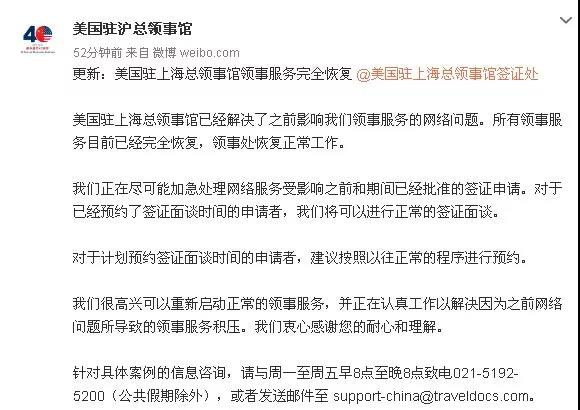# 上海美国使馆签证业务全面恢复

2019-09-04 11:20:36  |   来源：吾爱全球签证中心评论列表

（评论仅表达个人看法，并不表明本站同意其观点或证实其描述。）

### var day=""; var month=""; var ampm=""; var ampmhour=""; var myweekday=""; var year=""; mydate=new Date(); myweekday=mydate.getDay(); mymonth=mydate.getMonth()+1; myday= mydate.getDate(); year= mydate.getFullYear(); if(myweekday == 0) weekday=" 星期日 "; else if(myweekday == 1) weekday=" 星期一 "; else if(myweekday == 2) weekday=" 星期二 "; else if(myweekday == 3) weekday=" 星期三 "; else if(myweekday == 4) weekday=" 星期四 "; else if(myweekday == 5) weekday=" 星期五 "; else if(myweekday == 6) weekday=" 星期六 "; //document.write(year+"年"+mymonth+"月"+myday+"日 , "+weekday);

#### 编辑原创

GEOSTAR总裁赵凌云解析疫情后发展高端旅...

关注我们+